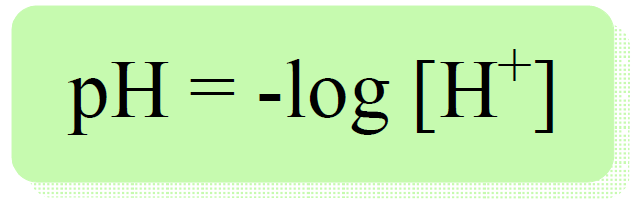## General Chemistry

In the previous post, we talked about pH and its measure for acidity.

Remember, pH is defined as the negative logarithm of proton/hydronium ion concentration:So, how do we know the concentration of proton or hydronium ion from the molarity of an acid?

Well, there are a few possibilities here depending on whether it is a strong or a weak acid. For strong acids such as HCl, HI, HClO4, HNO3, H2SO4 and etc, it is a relatively simple task because, for a strong acid, we assume a 100% dissociation, and therefore, the [H+] is the same as [HA].

Example: Calculate the pH of 2.49 M solution of HNO3.

HNO3 is completely ionized in water producing protons in a 1:1 molar ratio:

HNO3(aq) → H+(aq) +NO3(aq)

Therefore, [H+] = [HNO3] = 2.49 M, and

pH = -log 2.49 = 0.396

# The pH of a Strong Base

It is possible to calculate the pH from the concentration of a base.

For example, what is the pH of 0.450 M solution of NaOH?

The strategy here is to calculate the pOH and then, using the pH + pOH = 14 relationship, determine the pH.

NaOH is a strong base, so [OH] = [NaOH], and therefore, the pOH is:

pOH = -log 0.450 = 0.346

The pH then is equal to:

pH = 14 – pOH = 14 – 0.346 = 13.7

As expected, the pH is very high because it is a solution of a strong base.

In the next article, we will discuss determining the pH of a weak acid.

Check Also